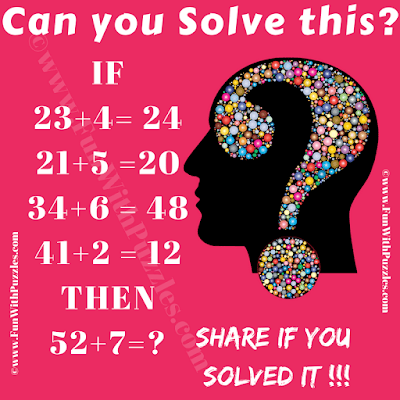It is challenging brain teaser for adults in which your challenge is to decipher the given logical equations. In the given Puzzle Picture, you are given some number equations. Find the logical pattern in these equations and then apply the same logical pattern in the last equation to find the value of the missing number which will replace the question mark. Do post your comment in case you are able to solve this challenging brain teaser.Can you solve this challenging brain teaser?